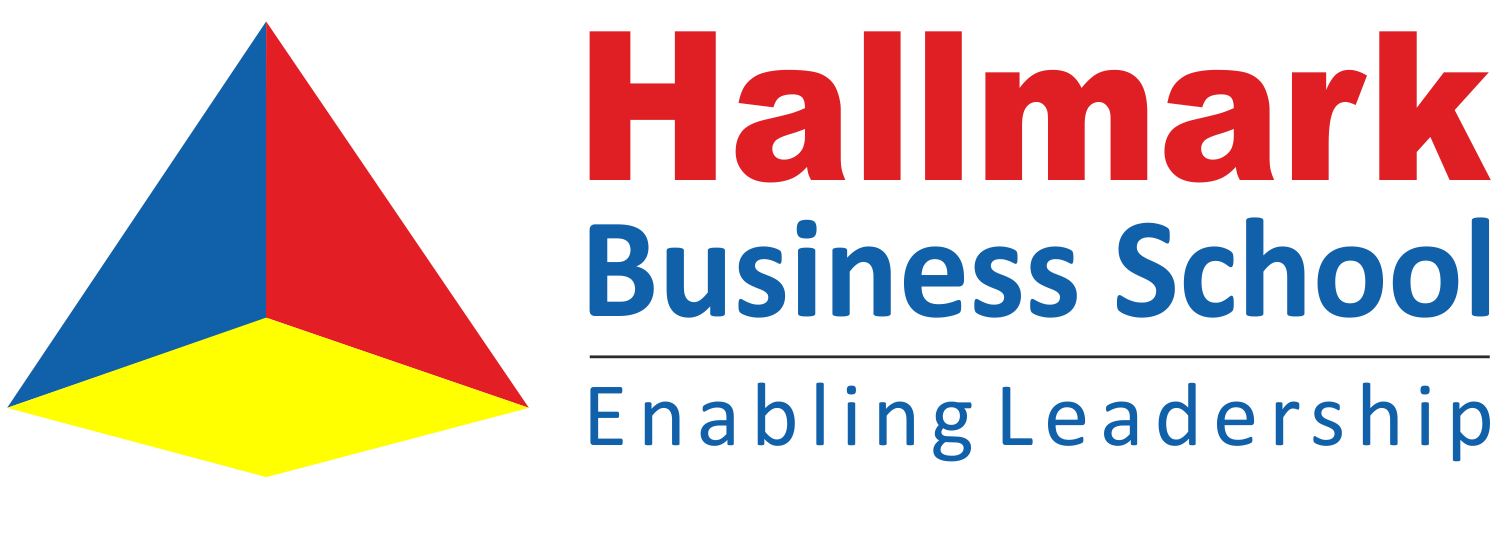# Statistics for Management### OBJECTIVE:

• To learn the applications of statistics in business decision making.

#### UNIT I - INTRODUCTION

Basic definitions and rules for probability, conditional probability independence of events, Baye‘s theorem, and random variables, Probability distributions: Binomial, Poisson, Uniform and Normal distributions.

#### UNIT II - SAMPLING DISTRIBUTION AND ESTIMATION

Introduction to sampling distributions, sampling distribution of mean and proportion,   application  of   central limit theorem, sampling techniques. Estimation: Point and Interval estimates for population parameters of large sample and small samples, determining the sample size.

#### UNIT III - TESTING OF HYPOTHESIS – PARAMETIRC TESTS

Hypothesis testing: one sample and two sample tests for means and proportions of large samples (z-test), one sample and two sample tests for means of small samples (t-test),  F-test  for two  sample  standard deviations. ANOVA one and two way

#### UNIT IV - NON-PARAMETRIC TESTS

Chi-square test  for  single  sample  standard  deviation. Chi-square tests for independence of  attributes and goodness of fit.  Sign test for paired data. Rank sum test. Kolmogorov-Smirnov – test for goodness of  fit, comparing two populations. Mann – Whitney U test and Kruskal Wallis test. One sample run test.

#### UNIT V - CORRELATION AND REGRESSION

Correlation – Coefficient of Determination – Rank Correlation – Regression – Estimation of Regression line – Method of Least Squares – Standard Error of estimate.

### Outcomes:

• To facilitate objective solutions in business decision making.
• To understand and solve business problems.
• To apply statistical techniques to data sets, and correctly interpret the results.
• To develop skill-set that is in demand in both the research and business environments.
• To enable the students to apply the statistical techniques in a work setting.

### Text Books

1. Richard I. Levin, David S. Rubin, Masood H.Siddiqui, Sanjay Rastogi, Statistics for Management,Pearson Education, 8th Edition, 2017.
2. Prem. S. Mann, Introductory Statistics, Wiley Publications, 9th Edition, 2015.
3. T N Srivastava and Shailaja Rego, Statistics for Management, Tata McGraw Hill, 3rd Edition 2017.
4. Ken Black, Applied Business Statistics, 7th Edition, Wiley India Edition, 2012.
5. David R. Anderson, Dennis J. Sweeney, Thomas A.Williams, Jeffrey D.Camm, James J.Cochran,Statistics for business and economics, 13th edition, Thomson (South – Western) Asia, Singapore,2016.
6. N. D. Vohra, Business Statistics, Tata McGraw Hill, 2017